1578493464

# All You Need to Know About JavaScript Functions

## Everything you ever needed to know about JavaScript functions.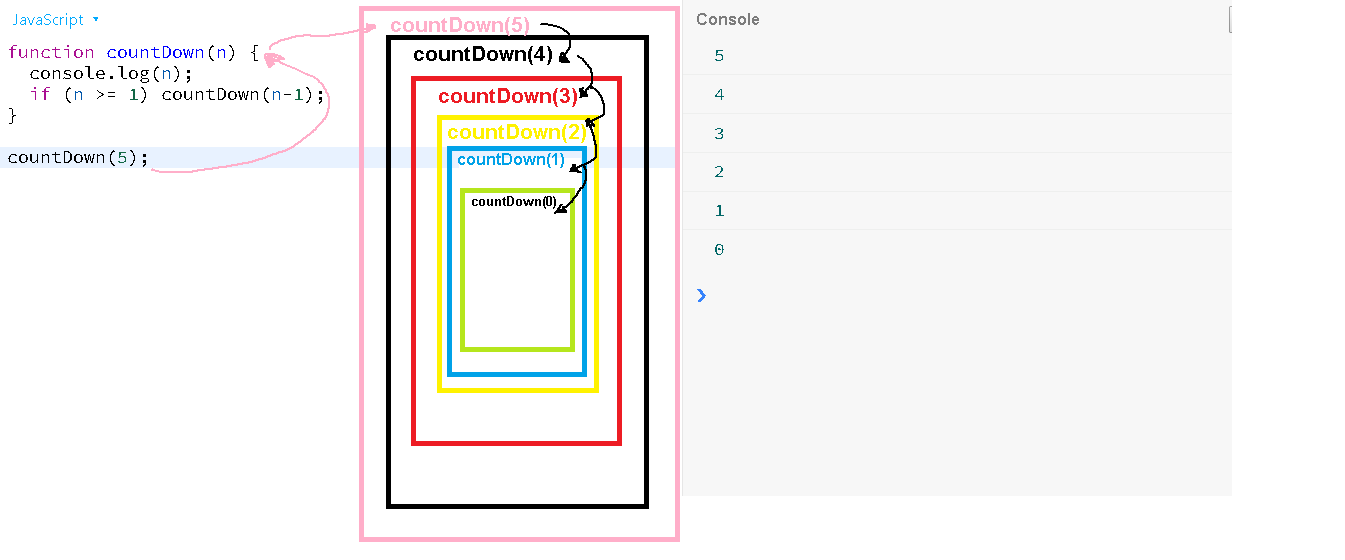Let’s begin at the beginning.

What is a function ?

Function, generally speaking, is a “subprogram” or a reusable piece of code that is designed to perform a particular task.

It is composed of a series of statements called function body. Values can be passed to a function and the function can/will return a value.

Now, with modern applications, functions can be a complete program in itself, rather than the general notion of a “subprogram”.

The layman differentiation between a function and a procedure is that the functions ideally should return a value and the procedure doesn’t (now this may vary according to the programming language under consideration).

As with everything lets write a ‘function’ that prints hello to console.

### Function that does not take a parameter and doesn’t return anything.

``````function sayHello () {
console.log("Hello !");
}
``````

The above function does not take a parameter and doesn’t return a value;

The above function can be called/invoked as follows:

``````sayHello();
``````

Now, you may prefer to use a semicolon or choose to omit it (as we will not get into the war of whether to use a semicolon or not to terminate a javascript statement.)

The output of the above code will be following in the console.If you are using es6/es2015 then the same function can be written as an arrow function.

``````const sayHello = () => {
console.log("Hello !");
}
``````

The arrow function is a concise way to write a function. It can be invoked exactly as before.

``````sayHello();
``````

An arrow function expression has a shorter syntax than a function expression and does not have its own this, arguments, super, or new.target (more about this later).

These function expressions are best suited for non-method
functions and they cannot be used as constructors.

What do I mean when I say, the above function doesn’t return anything?.

If I try to store the result of calling the function in a variable, it will be undefined.

For e.g.

``````let message = sayHello();

// The below console.log will return undefined as the function
// doesn't return any value.

console.log (message);
``````

### A function that does take a parameter but doesn’t return anything.

Let’s write a function that take one parameter but doesn’t return anything.

``````function log (message) {
console.log (message);
}
``````

The above function takes one parameter, named message, and logs the value to the console, and ends.

You can invoke the above function as shown below.

``````// The below call to log() function, logs the output to the
// and returns undefined.
log ("Hello JavaScript!");
``````

NOTE: If the function doesn’t return any value explicitly, then by default it returns “undefined”

### A function that takes a parameter and returns a value.

Let’s write a function that takes a number as a parameter and returns the square of the number.

``````function square(number) {
return number * number;
}
``````

console.log(square(2));

The output of the above function execution is shown below.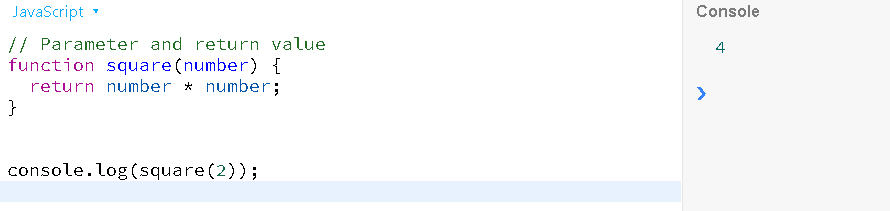### Functions are first class objects

Functions are first-class objects and they can be assigned to a variable and also be passed as a parameter. We will see an example of this.

But let’s see how to assign the above function into a variable and use it.

``````// You can also, use var or let.  I am using const indicating
// that this function cannot be reassigned once declared.

const square = function (number) {
return number * number;
}

console.log(square(2));
``````

Let’s write the above function as an arrow function.

``````const square = (number) => {
return number * number;
}

console.log(square(2)); // Outputs: 4
``````

### A function can take more than one arguments(in fact, it can take ’n’ parameter)

There is no theoretical maximum number of arguments/parameters in the spec but there are practical limits.

Stackoverflow: javascript functions, maximum no. of arguments

### How to write a function that can take ’n’ arguments?

Let’s write a sum() that can take ’n’ arguments and returns the sum of the arguments.

``````// Old way
const sum = function () {
let result = 0;
for(let i = 0; i < arguments.length; i++) {
result += arguments[i];
}
return result;
}
``````

We can invoke the above functions as shown below.

``````console.log(sum(1,2));
console.log(sum(1,2,3,4));
console.log(sum(1,3,5,7,9));
``````

### How does it work?

If you look at the sum function, it is not taking any parameter explicitly. Now imagine you are implementing this sum() function explicitly, how difficult it would be to define all the parameters upfront. You don’t know, with how many parameters the sum() function will be invoked.

It can be invoked with 1 parameter as sum(1) which returns 1.
It can be invoked with 2 parameters as sum(1,2) which returns 3.
It can be invoked with 100 parameters as sum(1,2,3,4,5,6…………,100) which returns 5050.

So, JavaScript provides us with this secret “arguments” object, that contains all the parameters and can be used within any functions.

Now note, the arguments object is not an array but an array-like object. that means you cannot invoke any array methods on the arguments object (More on this later. If you are too curious, do some research on this).

The working of the above sum() is pictorially shown below.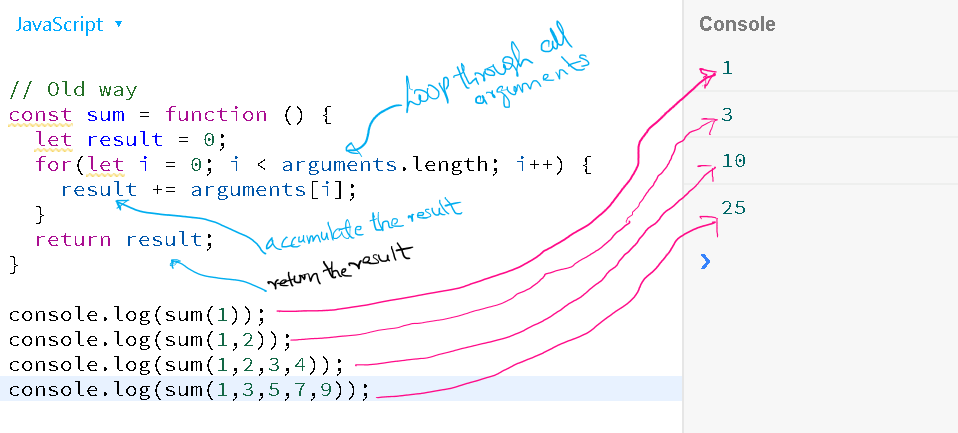When I say that the “arguments” is an array-like object, let’s see this in a picture with one invocation of the sum function. Observe the console.log of arguments. What do you see?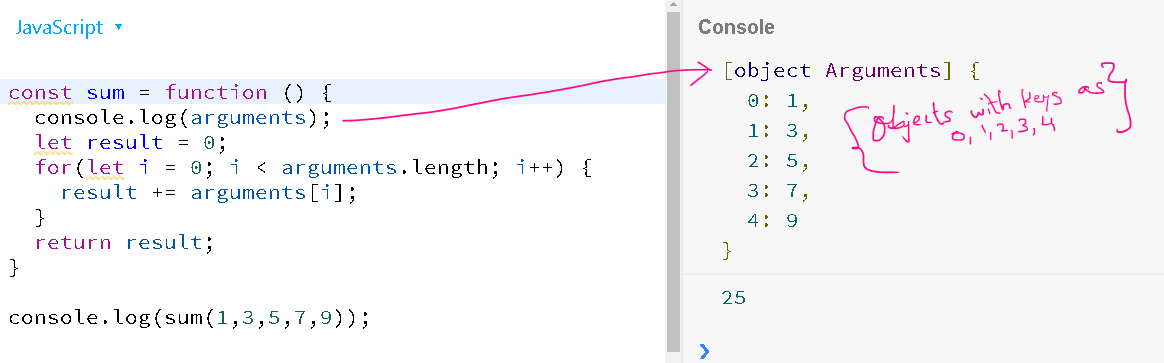In the above picture its very clear that the “arguments” is an object with the keys representing the index and the values, the actual parameter.

This object is like any other object. For e.g.

``````{
name:  "Rajesh",
hobbies: ["writing","programming"]
}

except the keys in the 'arguments' object looks like array index as
{
0:  "rajesh",
1:  ["writing","programming"]
}

Both of the above are object representation.
``````

NOTE: In modern JavaScript era using “arguments” object is not recommended. We can use a new concept called rest parameters.

Let’s see how to achieve the above result without using arguments object, but with REST parameters.

``````// New way using REST parameter
const sum = function (...args) {
let result = 0;
for(let i = 0; i < args.length; i++) {
result += args[i];
}
return result;
}
``````

Everything is same in the function except the magical “arguments” is replaced with the explicit REST parameter (…args). Now you can call it anything, here, as a convention, I choose to call it “args”.

### What does `...args does?`

The …args takes every parameter passed to the function and make it available as an actual array object. Remember I mentioned earlier that the “arguments” object is an array-like object and not an actual array, but the …args here is an actual array.

Let’s take one example of invoking the sum function again and also put a console.log statement in the sum function as shown below. This time we will use the `reduce` method of the array. As I mentioned earlier that …args are a real array we can use any method on it.

``````const sum = function (...args) {
console.log(args);
let result = 0;
result = args.reduce((current, prev) => {
return current + prev;
});
return result;
}

sum(1,2,3,4,5);
``````

The output of invoking the sum function along with the console.log(args) is shown below, as they say “A picture is worth a 1000 words”.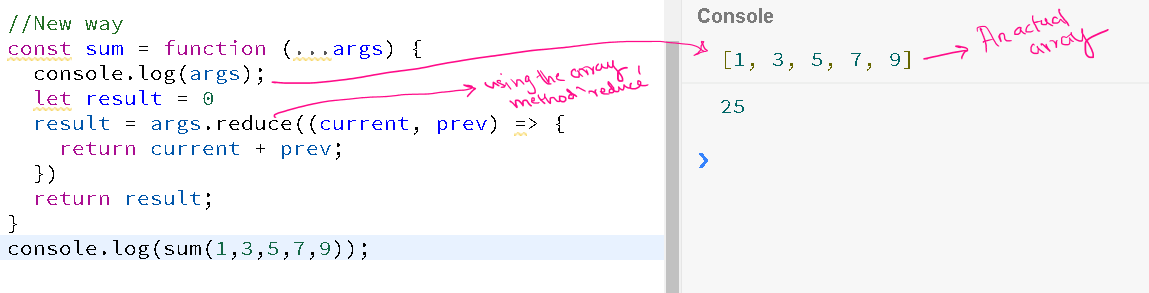In the above log, you can easily visualize the `…args` is an array. And since it is an array we can use the reduce method of the array to compute the sum.

NOTE: Explore the web, or watch out for my other article on understanding ‘reduce’ function.

…args can also be partially used. Lets take an example to complete this part.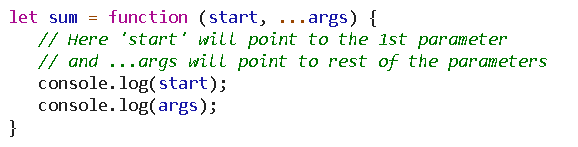Now if we invoke the sum function with three parameter(note: we are not doing actual summation) sum(1,2,3), and the output we get is,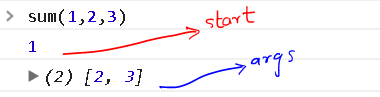### A function taking ‘function’ as a parameter

As we mentioned earlier in this article that a function is a first class object and hence it can also be passed as a parameter/argument to a function.

Let us write a function that takes a function as a parameter.

``````function dispatch (fn) {
fn();
}
``````

In the above code, we define a function named ‘dispatch’ which takes a function as an argument. Note the name ‘fn’ is just a convention, you can use any name. The other common name is ‘callback’ but used in a different context.

NOTE: We will use the term ‘callback’ when we are referring to functions that take function as a parameter.

Now, how to use the above function?

Let’s call/invoke the above function as shown below. You can use the normal function syntax or arrow function, whichever you prefer. I will use the arrow function notation.

``````METHOD 1: Defining function as a variable and passing it.
var fn = () => { console.log( "Hello !"); }
// Invoke the dispatch () function
dispatch(fn);  // Outputs "Hello !"

METHOD 2:  Defining an normal anonymous function inline.
dispatch (function () {
console.log("Hello !");
});

METHOD 3:  Defining an arrow function inline
dispatch (() => { console.log ("Hello !") });
``````

NOTE: All the above 3 methods of dispatching is the same.

The ‘callback’ function can also take a parameter and return value. Let’s see one more example of this.

``````function dispatch(fn) {    // Takes 'function' as an argument
return fn("hello");   // You can send some parameters.
}
``````

The above dispatch function takes a function as an argument and returns the return value from the passed in function. It invokes the passed in function with an argument as well.

How to invoke this function?

``````let result = dispatch(function (p1) {
return `My message and \${p1}`;
});
``````

Ponder over this for a moment.

### Practical applications of callback functions

Let’s say we have to execute some method after 1 second and not immediately. Here’ we can use the setTimeout function.

``````setTimeout(function () {
console.log('Check the status of some server...');
}, 1000);
``````

The above method will wait for minimum 1 second before executing. Please note, that the time passed to setTimeout and setInterval is in the millisecond and indicates the minimum time (but the minimum time is not guaranteed. More on that on a separate article).

What if you need to execute some operations after every 5 seconds. This is where setInterval comes into play.

``````// Here I am using the arrow function notation.
setInterval(()=> {
console.log("This will be executed every 5 second");
}, 5000);
``````

Within these functions you can write any code, may make some ajax calls etc.

NOTE: I am mixing arrow function notation and normal functions just so that by the time readers finishes this article they are comfortable with both approach.

You will find the callback method in real life in ajax calls, configuring routes for applications etc. Maybe I will write a separate article on that.

### A function can call itself (aka recursion)

Recursion is an interesting concept where a function calls itself. Now if you don’t handle the terminal condition, the function can run infinitely and finally the browser may vomit a stack overflow exception like ‘Maximum call stack size exceeded’.

Let us see what recursion looks like and a useful application of recursion.

``````function runForEver() {
runForEver();
}

// You can invoke the above function by running
runForEver();   // If you try you will get the call stack error
``````

The above function is a simple representation of a recursive function call. As the name suggests, this function will run forever, until the above error is thrown by the browser.

Ok, that’s a pretty useless function.

Let’s now write a little useful function for printing a countdown from a start value.

``````function countDown(n) {
console.log (n);
if (n >= 1) {  // Exit or terminal condition
countDown(n-1);
}
}
``````

You can invoke/run the above function by the code below.

``````countDown(5);  // -> The output will be 5, 4, 3, 2, 1
``````

How does this recursive function work?From the above diagram it is very clear how the recursive calls create a stack and in case we forgot to put the terminal condition then the stack will grow infinitely and finally you will be presented with the “Maximum call stack size exceeded” error.

Let us build a more practical example. Assume you have the below data structure.

``````let data = [
{
children :[
{
children: [
]
},
]
},
{
children :[
]
}
]
``````

Above, we have a hierarchical structure, that may be representing a menu or anything you wish.

We want to take this as an input and to create an unordered list with the correct hierarchy.

For eg., the final output should be as shown below.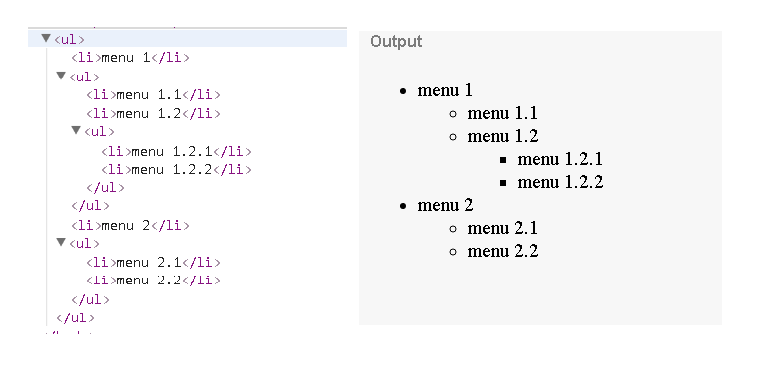Let’s code our recursive function that transforms the data into the above

pairs.

First, let’s see how we intend using the function.

``````let uls = buildTree(data);
// Output data to console
console.log(uls);

// Render on the window
// Only for demo. In real case append it to any parent element

document.write(uls);
``````

Let us now implement the buildTree function.

``````// Accepts two arguments
// data-> the data to transform
// isChild -> default false, used to indicate whether the node
//            being rendered is a child element or notfunction buildTree(data, isChild = false) {
let html = '<ul>';   // Let's initial html variable
// Run the forloop over the data
data.forEach((d) => {
// For every data element render an <li>
html += `<li>\${d.title}</li>`;
// If the current data element has children then call the
// buildTree again passing in the children and isChild = true
if (d.children) {
html += buildTree(d.children, true);
}
});
// Build the closing <ul> tag
html += '</ul>';
return html;  // Return the html
}
``````

### Immediately Invoked Function Expression aka IIFE

Let us now understand what an IIFE is and what problem does it solves. Before that let’s see what an anonymous function is.

An anonymous function is a function without a name. For e.g.

``````function () {
let message = "I don't have a name";
console.log(message);
}
``````

If the function doesn’t have a name, then how will you invoke it?

The answer is you can’t unless it is part of a callback function parameter.

Now, let’s see how to execute the above anonymous function.

``````(function () {
let message = "I don't have a name";
console.log(message);
})();
``````

Wrap the function within a parenthesis (this makes it an expression) and invoke it using a pair of opening and a closing parenthesis ().

The beauty of IIFE is that it can only be invoked once.

### Where can IIFE be used?

It can be used in scenarios where you need to run the function only once, like fetching some initial data, setting some configuration values, checking system status on startup etc.

### Technical Notes for the Nerdy among you

All functions are objects but not all objects are functions. What distinguishes the function from other objects is that functions can be called. In brief, they are `Function` objects.

### Objects and Functions (call, apply and bind)

Let’s have a look at how objects and functions can co-exist. Every function has access to three methods

• call
• apply
• bind

Let us see the usage of the above with an example. Let’s take the following object to work with.

``````let user = {
displayName: "rajesh",
sendMessage: function (message) {
console.log(`Sending \${message} to \${this.displayName}`);
}
}

let student = {
displayName: "rohan"
}
``````

The above code creates two variables for representing the user and student object. The astute reader might notice that the user object has some additional properties and method whereas the student object has only one property, and that matches with the user object.

Let us see how to send a message to the user. It’s very easy as dropping in the code below.

``````user.sendMessage("Hello...");
``````

And the message “Hello…” will be logged to console.

Please note within the function sendMessage() the ‘this’ refers to the object on which the function was invoked, in this case, the `user` object.

Now, how can you call the sendMessage() on the student object (a weird scenario but sometimes useful in many practical cases).

This is where the call, apply and bind comes into action.

### Using call() method to invoke a method on another object

``````user.sendMessage.call(student, “Hello from Rajesh”);
``````

The first parameter to call method is the new context, in this case, student, and the subsequent parameters are the arguments for the sendMessage, in this case, the text ‘Hello from Rajesh’;

This will print the output ‘Hello from Rajesh to Rohan’. Within sendMessage, the `this` will now point to the `student` object rather than the `user`object.

### Using apply() method to invoke a method on another object

``````user.sendMessage.apply(student, [“Hello from Rajesh agin..”]);
``````

The first parameter to apply method is the new context, in this case, student, and the subsequent parameters are the arguments for the sendMessage, in this case, the text ‘Hello from Rajesh’;

In apply() method the arguments are passed as an array. This is the only difference between call() and apply()

This will print the output ‘Hello from Rajesh again… to Rohan’. Within sendMessage, the `this` will now point to the `student` object rather than the `user`object.

### Using bind() method

The bind method returns a new method with the new context. For e.g. let’s create a new variable that will point to the sendMessage() but with the new context.

``````let sendMessageToStudent = user.sendMessage.bind(student);
``````

The above line creates a new variable `sendMessageToStudent` which is actually a function which you can invoke. But the `this` context will point to `student` object rather than `user` object.

You can invoke the function as shown below.

``````sendMessageToStudent("Yet another message");
``````

This will output the text ‘Sending Yet another message… to Rohan”.

I hope this makes the concepts of call, apply and bind very clear.

### Constructor Function

A constructor function in JavaScript starts with an Uppercase letter by convention. It is a mechanism via which we can simulate object-oriented programming using JavaScript.

A Constructor function is invoked by the `new` keyword. You can pass parameters to constructor function just like any other function and it can also return object.

NOTE: If you return a simple value like `string` or `number` or `boolean` from a constructor function, it will be ignored and the instance of itself will be return.

Let’s create a constructor function representing a `User` object.

``````function User (name, email) {
this.name = name;   // instance variables
this.email = email;

// You can define methods here, but is not recommended
// from performance perspective.
// See the prototype method below
this.save = function () {
// Do whatever you want
this.id = +new Date();
//console.log(`\${this.name} saved to DB successfully!`);
return this.id;
}
}

// PROTOTYPE: Recommended way to create instance methods
User.prototype.saveDB = function () {
this.id = +new Date();
//console.log(`\${this.name} saved to DB successfully!`);
return this.id;
}
``````

So, the above constructor function represents a template for a `User` object. It takes name and email as an argument and assigns it to the newly created instance (when someone invokes it with the `new` keyword).

You can also add methods to the function directly on `this`, but it is not recommended for scenarios where you will be creating a lot of objects. For one of instance or singleton cases this will work fine (more on that later).

Within the constructor function the `this` points to the current instance. Please note just after the function we are also creating a duplicate method on the function’s prototype.

Before we go into detail the pros and cons of creating methods directly on `this` vs `prototype`, let see the output of this function and how this can be invoked.

``````let user = new User('rajesh', 'someemail@test.com');
console.log(user);
console.log(user.save());
console.log(user.saveDB());  // Exact output as save().

// Access instance variables/methods
console.log(user.email);  // Outputs emails
``````

So, the above code creates an instance of a User object and invokes the save() method. Within the constructor function, you can access the instance variables by using `this.variablename`.

The output on the console will be as shown below.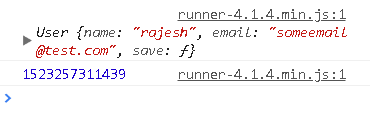As a convention, functions inside the constructor function or on the prototype is called as `methods` just to avoid any confusion.

NOTE: If you fail to use the ‘new’ keyword with User, the function will not work as expected as inside the function ‘this’ will point to global object.

So, the below code will not work.

``````// WILL NOTE WORK.  YOU HAVE TO USE 'new' with Constructor function
let user = User("rajesh","someemail2@test.com");
``````

### Why use a prototype for adding instance methods?

Now, we know both the `save()` and `saveDB()` method above works similarly, why use prototype.

Let’s take the below scenario.

Use Case: Read users list from external file / API and create user objects to work within the application.

Since we are not dealing with external API/web services here, let’s create 100 users in a loop.

``````let users = [];
for(let i = 1; i <= 100; i++) {
let user = new User(`user \${i}`);
user.email = `user\${i}@test.com`; // Lets create dynamic email
users.push(user);
}

console.log(users);
``````

Now let’s take a look at the `users` array.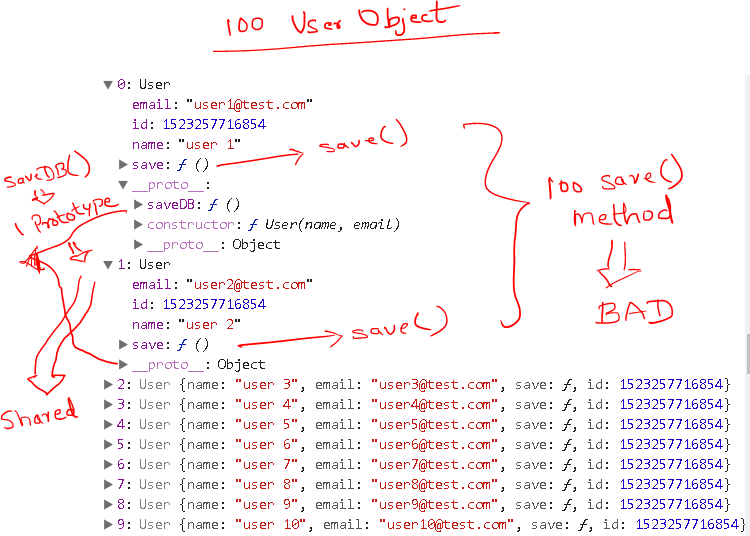Observe the array. There are 100 user objects and 100 copies of the `save()` method . This is very BAD for memory.

Whereas’ the `saveDB()` is attached only once to the prototype of `User`. And any method added to the prototype is shared by all instances of the constructor function, in this case `User`.

### Providing protection against missing ‘new’ for invoking constructor function.

What if you want to provide protection against missing ‘new’ when invoking constructor function.

For e.g., you want both the below code to work.

``````let user1 = new User("rajesh", "somemail2@test.com");
let user2 = User("rohan", "somemail3@test.com");
``````

We can put a simple guard condition in the constructor function as shown below to achieve the desired result. Please modify this as per your requirement. In some cases, you will prefer to throw an exception.

I am only shown the part code of User function.

``````function User (name, email){
if (!(this instanceof User)) {
return new User(name, email);  // Don't forget parameter if any
}

// REST OF THE CODE GOES HERE

}
``````

So, the above takes care of missing new for constructor function. We are checking if the instance is not of type User then create a new User object with the required parameters and return it.

In case the above code is invoked as

``````let user = User("rajesh","someemail@test.com");
``````

It will correctly return the instance of User object.

### How to return a custom object from a Constructor function?

As we discussed earlier we can return any object from constructor function except primitive types.

For example, take a look at the below code.

``````function Api(baseUrl) {
let _secret = +new Date();
let self = this;  // in case you access to 'this' of Api.  return {
fetchData: function (resource) {
// Here you cannot use 'this' as 'this' points to the
// fetchData function.      // The 'self' variable created above will point the the
// API instance
let url = `\${baseUrl}/\${resource}/`;
console.log(url);
fetch(`\${url}`)
.then(response => response.json())
.then(json => console.log(json));
}
}
}
``````

The above approach is typically used for singleton pattern(we will cover Singleton pattern in a while), where you only need one instance to work with. You can also use the object literal syntax to create this type of behavior.

Now, let’s see how to use the above function. I am using the free json service from typicode.

``````let api = new Api("https://jsonplaceholder.typicode.com");
api.fetchData("posts");   // Get posts data

api.fetchData("users");  // Get users data
``````

One gain that you get from the above approach is that even if you miss the `new` keyword the function will work correctly as you are explicitly returning the object and you are not relying on the `this` context.

For e.g. the above code can also be written as

``````let api = Api("https://jsonplaceholder.typicode.com");
api.fetchData("posts");   // Get posts data
``````

### Singleton

Singleton is a design pattern wherein you can have only one instance of a class or a constructor function.

We can easily create a single object by using IIFE. IIFE is executed only once and hence we can control the creation of our objects by wrapping our singleton object within IIFE and returning a new instance or an existing instance as required.

``````var Singleton = (function () {
var instance;

function createInstance() {
var object = new Object("I am the instance");
return object;
}

return {
getInstance: function () {
if (!instance) {
instance = createInstance();
}
return instance;
}
};
})();
``````

We can use the above function as shown below.

``````let instance1 = Singleton.getInstance();
let instance2 = Singleton.getInstance();

console.log(instance1 === instance2);   // Will return true
``````

How does Singleton work?

We first wrap the entire code in an IIFE and create a local variable named `instance`. In the getInstance() method, we check if the local variable is already initialized. If no, we create a new instance using the createInstance() function otherwise we return the already created instance.

Now, no matter how many time you call getInstance(), you will only get one copy of the object in memory. This is a very useful pattern for conserving memory and for creating utility functions where only one instance is required.

## Closures

A closure is the combination of a function and the lexical environment within which that function was declared.

The word “lexical” refers to that fact that lexical scoping uses the location where a variable was declared within the source code to determine where that variable is available.

### Applications of Closures

Partial Application
The process of applying a function to some of its arguments. The partially applied function gets returned for later use.

In other words, a function that takes a function with multiple parameters and returns a function with fewer parameters.

Event Handlers

Ajax

Private methods

Let us see a very simple example of closure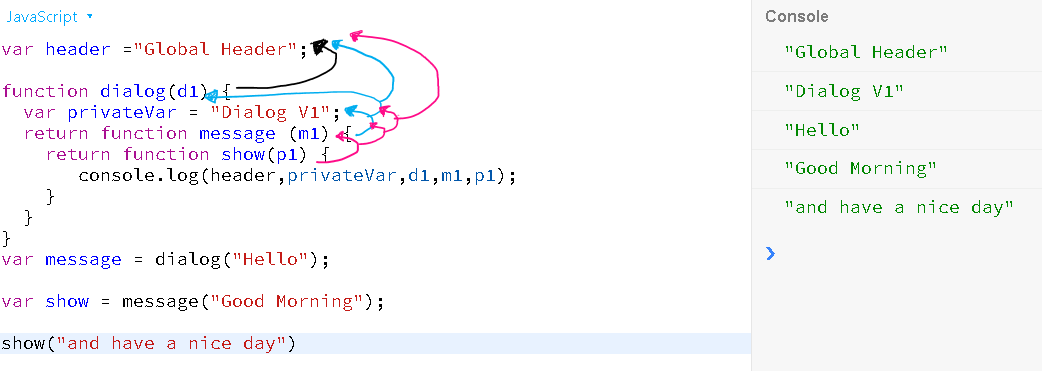In the above functions let’s see what each function has access to.

The message () has access to its parameter, m1, and its parent function, dialog()’s d1 parameter. It also has access to the `privateVar.`

The show () has access to its parameter, p1, its parent function scope, message, and the variables from the dialog function.

Finally, when the show() function is invoked it has access to all the parameters enclosed by it, even though, those variables and parameters were not in scope when the show method is executed.

This is closure. It encloses all the surrounding variables and functions long after they are out of scope.

#javascript #web-development

## Buddha Community1605017502

## The Difference Between Regular Functions and Arrow Functions in JavaScript

Other then the syntactical differences. The main difference is the way the this keyword behaves? In an arrow function, the this keyword remains the same throughout the life-cycle of the function and is always bound to the value of this in the closest non-arrow parent function. Arrow functions can never be constructor functions so they can never be invoked with the new keyword. And they can never have duplicate named parameters like a regular function not using strict mode.

###### Here are a few code examples to show you some of the differences
```this.name = "Bob";
const person = {
name: “Jon”,
```<span style="color: #008000">// Regular function</span>
func1: <span style="color: #0000ff">function</span> () {
console.log(<span style="color: #0000ff">this</span>);
},

<span style="color: #008000">// Arrow function</span>
func2: () =&gt; {
console.log(<span style="color: #0000ff">this</span>);
}
```
}
person.func1(); // Call the Regular function
// Output: {name:“Jon”, func1:[Function: func1], func2:[Function: func2]}
person.func2(); // Call the Arrow function
// Output: {name:“Bob”}```

###### The new keyword with an arrow function
```const person = (name) => console.log("Your name is " + name);
const bob = new person("Bob");
// Uncaught TypeError: person is not a constructor```

###### If you want to see a visual presentation on the differences, then you can see the video below:

#arrow functions #javascript #regular functions #arrow functions vs normal functions #difference between functions and arrow functions1601069940

## Function Expression vs Function Declaration in JavaScript

Function Expression vs Function Declaration in JavaScript.

It was until during one of the JavaScript mock interviews did I came across the term function expression.

The question was: What is the difference between these two syntax?

function x(){

}

let x = function(){

}

I was clueless for a moment. After thinking a little, I could only come up with: the second syntax invokes an _anonymous _function and is assigned to a variable.

I was alien to the term hoisting.

In this article, we will acquaint ourselves with three simple terms: function declaration,_ function expression, _and hoisting.

What is function declaration?

Function declaration is also known as _function statement. _It contains the name of the function, parameters, and a return statement. **Naming the function **is what sets function declaration apart. Parameters and return statement is optional.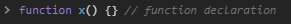Function Declaration

What is function expression?

Function expression also has a name, parameters, and return statement. All of which are optional. The important thing to bear in mind is: the function here is _assigned _to a JavaScript variable.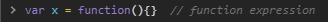Function Expression

#function-expression #function-declaration #functions-in-javascript #coding #javascript #express1624410000

## JavaScript Factory Functions

📺 The video in this post was made by Programming with Mosh
The origin of the article: https://www.youtube.com/watch?v=jpegXpQpb3o&list=PLTjRvDozrdlxEIuOBZkMAK5uiqp8rHUax&index=9
🔥 If you’re a beginner. I believe the article below will be useful to you ☞ What You Should Know Before Investing in Cryptocurrency - For Beginner
⭐ ⭐ ⭐The project is of interest to the community. Join to Get free ‘GEEK coin’ (GEEKCASH coin)!
Thanks for visiting and watching! Please don’t forget to leave a like, comment and share!

#javascript #factory functions #functions #javascript factory functions1624392000

## JavaScript Constructor Functions. IN JUST 6 MINUTES!!!

📺 The video in this post was made by Programming with Mosh
The origin of the article: https://www.youtube.com/watch?v=23AOrSN-wmI&list=PLTjRvDozrdlxEIuOBZkMAK5uiqp8rHUax&index=10
🔥 If you’re a beginner. I believe the article below will be useful to you ☞ What You Should Know Before Investing in Cryptocurrency - For Beginner
⭐ ⭐ ⭐The project is of interest to the community. Join to Get free ‘GEEK coin’ (GEEKCASH coin)!
Thanks for visiting and watching! Please don’t forget to leave a like, comment and share!

#javascript #functions #constructor #constructor functions #javascript constructor functions1603176407

## The real reason why JavaScript has arrow functions

Nowadays, all my code is based on the use of arrow functions. If you are still not using them yourself, then don’t be ashamed of who you are. That’s your parent’s job. Instead, find about all the benefits that you can get by using arrow functions like the cool kids.

This is an example of arrow function and the same code written traditionally:

``````const arrowFunction = (arg1, arg2) => arg1 + arg 2;

const traditionalFunction = function(arg1, arg2) {
return arg1 + arg2;
};
``````

You may notice that the code is shorter and that there is an arrow. Everything before the arrow is arguments of the function and everything after the arrow is always returned as the result of the function.

If you need a function that contains multiple statements you can still do this:

``````const arrowFunction = (arg1, arg2) => {
const result = arg1 + arg2;
return result;
};
``````

#javascript #js #functional-javascript #functional-programming #javascript-tips